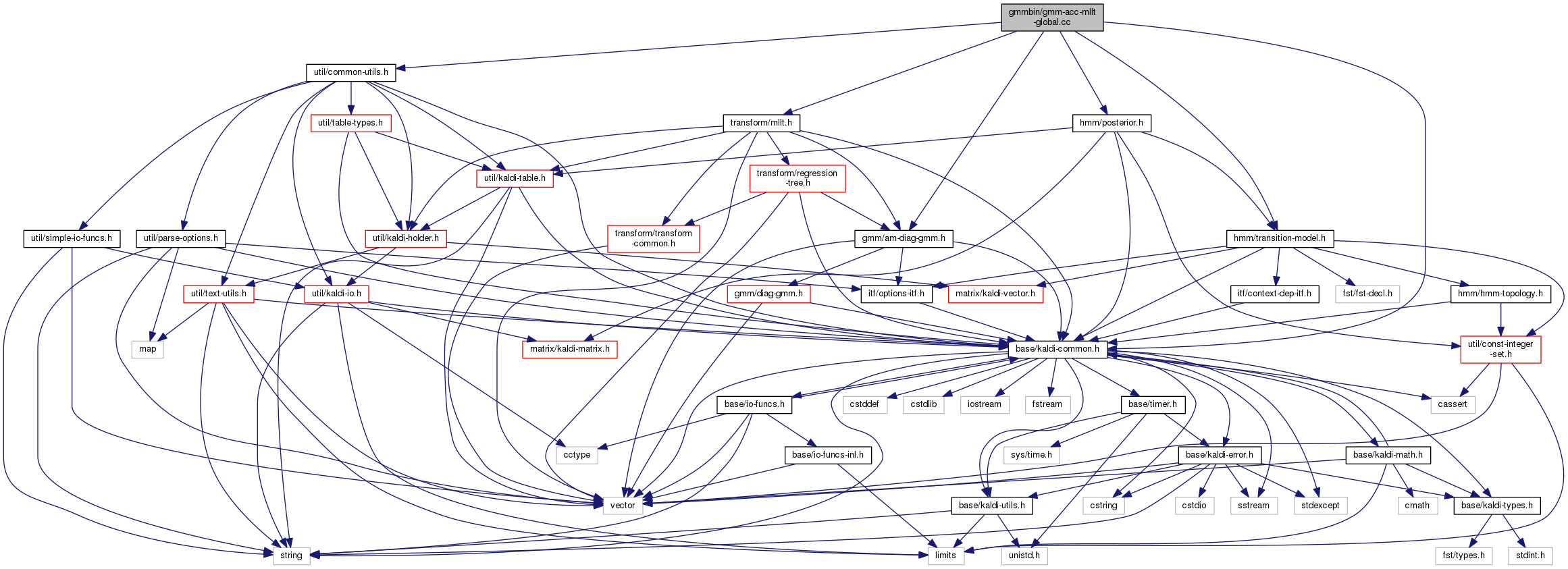gmm-acc-mllt-global.cc File Reference
`#include "base/kaldi-common.h"`
`#include "util/common-utils.h"`
`#include "gmm/am-diag-gmm.h"`
`#include "hmm/transition-model.h"`
`#include "transform/mllt.h"`
`#include "hmm/posterior.h"`
Include dependency graph for gmm-acc-mllt-global.cc:Go to the source code of this file.

## Functions

int main (int argc, char *argv[])

## ◆ main()

 int main ( int argc, char * argv[] )

Definition at line 31 of file gmm-acc-mllt-global.cc.

31  {
32  using namespace kaldi;
33  try {
34  const char *usage =
35  "Accumulate MLLT (global STC) statistics: this version is for where there is\n"
36  "one global GMM (e.g. a UBM)\n"
37  "Usage: gmm-acc-mllt-global [options] <gmm-in> <feature-rspecifier> <stats-out>\n"
38  "e.g.: \n"
39  " gmm-acc-mllt-global 1.dubm scp:feats.scp 1.macc\n";
40
41  ParseOptions po(usage);
42  bool binary = true;
43  BaseFloat rand_prune = 0.25;
44  std::string gselect_rspecifier;
45  po.Register("binary", &binary, "Write output in binary mode");
46  po.Register("rand-prune", &rand_prune, "Randomized pruning parameter to speed up "
47  "accumulation (larger -> more pruning. May exceed one).");
48  po.Register("gselect", &gselect_rspecifier, "Rspecifier for Gaussian selection "
49  "information");
51
52  if (po.NumArgs() != 3) {
53  po.PrintUsage();
54  exit(1);
55  }
56
57  std::string gmm_filename = po.GetArg(1),
58  feature_rspecifier = po.GetArg(2),
59  accs_wxfilename = po.GetArg(3);
60
61  using namespace kaldi;
62  typedef kaldi::int32 int32;
63
64  DiagGmm gmm;
66
67  MlltAccs mllt_accs(gmm.Dim(), rand_prune);
68
69  double tot_like = 0.0;
70  double tot_t = 0.0;
71
74
75  int32 num_done = 0, num_err = 0;
78  const Matrix<BaseFloat> &mat = feature_reader.Value();
79
80  num_done++;
81  BaseFloat tot_like_this_file = 0.0, tot_weight = 0.0;
82
83  if (gselect_rspecifier == "") {
84  for (int32 i = 0; i < mat.NumRows(); i++) {
85  tot_like_this_file += mllt_accs.AccumulateFromGmm(gmm, mat.Row(i), 1.0);
86  tot_weight += 1.0;
87  }
88  } else {
90  KALDI_WARN << "No gselect information for utterance " << utt;
91  num_err++;
92  continue;
93  }
94  const std::vector<std::vector<int32> > &gselect= gselect_reader.Value(utt);
95  if (static_cast<int32>(gselect.size()) != mat.NumRows()) {
96  KALDI_WARN << "Gselect information has wrong size for utterance "
97  << utt << ", " << gselect.size() << " vs. "
98  << mat.NumRows();
99  num_err++;
100  continue;
101  }
102
103  for (int32 i = 0; i < mat.NumRows(); i++) {
104  tot_like_this_file += mllt_accs.AccumulateFromGmmPreselect(
105  gmm, gselect[i], mat.Row(i), 1.0);
106  tot_weight += 1.0;
107  }
108  }
109  KALDI_LOG << "Average like for this file is "
110  << (tot_like_this_file/tot_weight) << " over "
111  << tot_weight << " frames.";
112  tot_like += tot_like_this_file;
113  tot_t += tot_weight;
114  if (num_done % 10 == 0)
115  KALDI_LOG << "Avg like per frame so far is "
116  << (tot_like/tot_t);
117  }
118
119  KALDI_LOG << "Done " << num_done << " files. ";
120
121  KALDI_LOG << "Overall avg like per frame (Gaussian only) = "
122  << (tot_like/tot_t) << " over " << tot_t << " frames.";
123
124  WriteKaldiObject(mllt_accs, accs_wxfilename, binary);
125  KALDI_LOG << "Written accs.";
126  return (num_done != 0 ? 0 : 1);
127  } catch(const std::exception &e) {
128  std::cerr << e.what();
129  return -1;
130  }
131 }
This code computes Goodness of Pronunciation (GOP) and extracts phone-level pronunciation feature for...
Definition: chain.dox:20
int32 Dim() const
Returns the dimensionality of the Gaussian mean vectors.
Definition: diag-gmm.h:74
kaldi::int32 int32
void ReadKaldiObject(const std::string &filename, Matrix< float > *m)
Definition: kaldi-io.cc:832
Allows random access to a collection of objects in an archive or script file; see The Table concept...
Definition: kaldi-table.h:233
float BaseFloat
Definition: kaldi-types.h:29
The class ParseOptions is for parsing command-line options; see Parsing command-line options for more...
Definition: parse-options.h:36
const SubVector< Real > Row(MatrixIndexT i) const
Return specific row of matrix [const].
Definition: kaldi-matrix.h:188
A templated class for reading objects sequentially from an archive or script file; see The Table conc...
Definition: kaldi-table.h:287
#define KALDI_WARN
Definition: kaldi-error.h:150
MatrixIndexT NumRows() const
Returns number of rows (or zero for empty matrix).
Definition: kaldi-matrix.h:64
Definition for Gaussian Mixture Model with diagonal covariances.
Definition: diag-gmm.h:42
void WriteKaldiObject(const C &c, const std::string &filename, bool binary)
Definition: kaldi-io.h:257
#define KALDI_LOG
Definition: kaldi-error.h:153
A class for estimating Maximum Likelihood Linear Transform, also known as global Semi-tied Covariance...
Definition: mllt.h:42